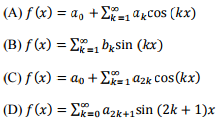# GATE 2016 7th Feb Solution/ Key : Electrical

Q.6 Consider the function ݂f(z)= z+z where z is a complex variable and z denotes its complex conjugate. Which one of the following is TRUE?
(A) ݂f(z) is both continuous and analytic
(B) ݂f(z) is continuous but not analytic
(C) ݂f(z) is not continuous but is analytic
(D) f(z) is neither continuous nor analytic

Q.7 A 3 × 3 matrix P ܲ is such that, P3 = P. Then the eigenvalues of ܲ are
(A) 1, 1, −1
(B) 1, 0.5 + j݆0.866, 0.5 − ݆j0.866
(C) 1,−0.5 + j݆0.866, −0.5 − ݆j0.866
(D) 0, 1, −1

Q.8 The solution of the differential equation, for t>0, y"(t) + 2y'(t) + y(t) = 0  with initial
conditions y(0)=0, and y'(0)= 1, is u(t) denotes the unit step function

Q.9 The value of the line integralalong a path joining the origin (0, 0, 0) and the point (1, 1, 1) is
(A) 0
(B) 2
(C) 4
(D) 6

Q.10 Let f(x) be a real, periodic function satisfying ݂f(-x)= -f(x). The general form of its Fourier series representation would bePlease report if you find any errors in the questions.Thanks for reading GATE 2016 7th Feb Solution/ Key : Electrical

←Previous
« Prev Post
Next→
Next Post »

1.2.